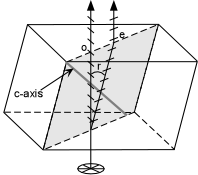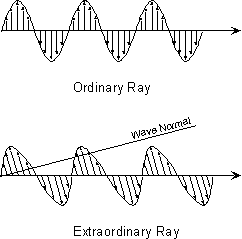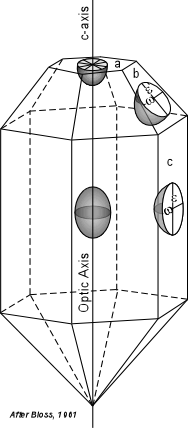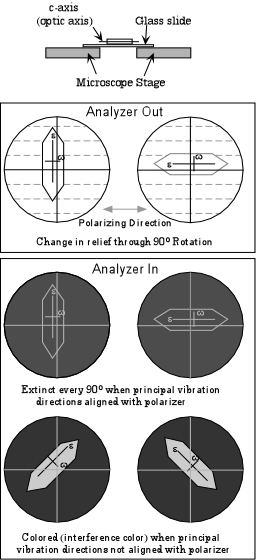EENS 2110 Mineralogy Tulane University Prof. Stephen A. Nelson Uniaxial Minerals, Uniaxial Indicatrix, Optic Sign, & Ray Path

 Introduction to Uniaxial Minerals Uniaxial minerals are a class of anisotropic minerals that include all minerals that crystallize in the tetragonal and hexagonal crystal systems.  They are called uniaxial because they have a single optic axis.  Light traveling along the direction of this single optic axis exhibits the same properties as isotropic materials in the sense that the polarization direction of the light is not changed by passage through the crystal.  Similarly, if the optic axis is oriented perpendicular to the microscope stage with the analyzer inserted, the grain will remain extinct throughout a 360o rotation of the stage.  The single optic axis is coincident with the c-crystallographic axis in tetragonal and hexagonal minerals.  Thus, light traveling parallel to the c-axis will behave as if it were traveling in an isotropic substance because, looking down the c-axis of tetragonal or hexagonal minerals one sees only equal length a-axes, just like in isometric minerals. Like all anisotropic substances, the refractive indices of uniaxial crystals varies between two extreme values.  For uniaxial minerals these two extreme values of refractive index are defined as ω (or No) and ε (or Ne).  Values between ω and e are referred to as ε'.   Uniaxial minerals can be further divided into two classes.  If ω > ε the mineral is said have a negative optic sign or is uniaxial negative.  In the opposite case, where ε > ω the mineral is said to have a positive optic sign or is uniaxial positive.  The absolute birefringence of a uniaxial minerals is defined as | ω - ε | (the absolute value of the difference between the extreme refractive indices).
 Double Refraction All anisotropic minerals exhibit the phenomenon of double refraction.  Only when the birefringence is very high, however, is it apparent to the human eye.  Such a case exists for the hexagonal (and therefore uniaxial) mineral calcite.  Calcite has rhombohedral cleavage which means it breaks into blocks with parallelogram - shaped faces.  If a clear rhombic cleavage block is placed over a point and observed from the top, two images of the point are seen through the calcite crystal.  This is known as double refraction. What happens is that when unpolarized light enters the crystal from below, it is broken into two polarized rays that vibrate perpendicular to each other within the crystal.
 One ray, labeled o in the figure shown here, follows Snell's Law, and is called the ordinary ray, or o-ray.  It has a vibration direction that is perpendicular to the plane containing the c-axis and the path of the ray.  The other ray, labeled e in the figure shown here, does not follow Snell's Law, and is therefore referred to as the extraordinary ray, or e-ray.  The e ray is polarized with light vibrating within the plane containing the c-axis and the propagation path of the ray.Since the angle of incidence of the light is 0o, both rays should not be refracted when entering the crystal according to Snell's Law, but the e-ray violates this law because it's angle of refraction is not 0o, but is r, as shown in the figure. Note that the vibration directions of the e-ray and the o-ray are perpendicular to each other.  These directions are referred to as the privileged directions in the crystal.
 If one separates out the e-ray and the o-ray as shown here, it can be seen that the o-ray has a vibration direction that is perpendicular to the propagation direction.  On the other hand, the vibration direction of the e-ray is not perpendicular to the propagation direction.  A line drawn that is perpendicular to the vibration direction of the e-ray is called the wave normal direction.  It turns out the wave normal direction does obey Snell's Law, as can be seen by examining the diagram of the calcite crystal shown above.  In the case shown, the wave normal direction would be parallel to the o-ray propagation direction, which is following Snell's Law.Uniaxial Indicatrix  Just like in isotropic minerals, we can construct an indicatrix for uniaxial minerals.  The uniaxial indicatrix is constructed by first orienting a crystal with its c-axis vertical.  Since the c-axis is also the optic axis in uniaxial crystals, light traveling along the c-axis will vibrate perpendicular to the c-axis and parallel to the ω refractive index direction.   Light vibrating perpendicular to the c-axis is associated with the o-ray as discussed above.  Thus, if vectors are drawn with lengths proportional to the refractive index for light vibrating in that direction, such vectors would define a circle with radius ω.  This circle is referred to as the circular section of the uniaxial indicatrix.
 Light propagating along directions perpendicular to the c-axis or optic axis is broken into two rays that vibrate perpendicular to each other.  One of these rays, the e-ray vibrates parallel to the c-axis or optic axis and thus vibrates parallel to the ε refractive index.  Thus, a vector with length proportional to the ε refractive index will be larger than or smaller than the vectors drawn perpendicular to the optic axis, and will define one axis of an ellipse.  Such an ellipse with the ε direction as one of its axes and the ω direction as its other axis is called the Principal Section of the uniaxial indicatrix.   Light vibrating parallel to any direction associated with a refractive index intermediate between ε and ω will have vector lengths intermediate between those of ε and ω and are referred to as ε' directions.Thus, the uniaxial indicatrix is seen to be an ellipsoid of revolution.  Such an ellipsoid of revolution would be swept out by rotating the ellipse of the principal section by 180o.  Note that there are an infinite number of principal sections that would cut the indicatrix vertically.  Light propagating along one of the ε' directions is broken into two rays, one vibrates parallel to an ε' direction and the other vibrates parallel to the ω direction.  An ellipse that has an ε' direction and a ω direction as its axes is referred to as a random section of the indicatrix.
 Optic Sign Recall that uniaxial minerals can be divided into 2 classes based on the optic sign of the mineral.
 If ω > ε, the optic sign is negative and the uniaxial indicatrix would take the form of an oblate spheroid.  Note that such an indicatrix is elongated in the direction of the stroke of a minus sign. If ε > ω, the optic sign is positive and the uniaxial indicatrix would take the form of a prolate spheroid.  Note that such an indicatrix is elongated in the direction of the vertical stroke of a plus sign.Application of the Uniaxial Indicatrix The uniaxial indicatrix provides a useful tool for thinking about the vibration directions of light as it passes through a uniaxial crystal.  Just like crystallographic axes, we can move the indicatrix anywhere in a crystal so long it is moved parallel to itself.
 This is shown here for an imaginary tetragonal crystal. In this case the optic sign of the mineral is positive, and the uniaxial indicatrix is shown at the center of the crystal.   If the crystal is mounted on the microscope stage such that the c-axis or optic axis is perpendicular to the stage, we can move the indicatrix up to the top face of the crystal (face a) and see that such light will be vibrating in the ω direction even if we rotate the stage.  Thus we will see the circular section of the indicatrix.  If the crystal is mounted on the stage such that the c-axis is parallel to the stage, we can move the indicatrix to one of the side faces of the crystal (such as face c) and see that light will be broken up into two rays, one vibrating parallel to the ε direction and one vibrating parallel to the ω direction.  Thus we will see one of the principal planes of the indicatrix.If the crystal is mounted on the stage such that the c-axis or optic axis is neither parallel to or perpendicular to the stage, we can move the indicatrix to some random face that is not parallel to or perpendicular to the c-axis (such as face b) and see that the light will be broken into two perpendicular rays, one vibrating parallel to the ω direction and the other vibrating perpendicular to an ε'  direction.  Thus we will see one of the random sections of the indicatrix. We will next look at what we could observe for crystals oriented on the microscope stage for each of the general orientations described above, beginning with the unique circular section.
 Circular Section If a crystal is mounted on the microscope stage with its optic axis oriented exactly perpendicular to the stage, the circular section of the indicatrix can be imagined to be on the upper surface of the crystal such as for the crystal face labeled a in the diagram above. In this orientation the crystal behaves just like an isotropic mineral.
 Light polarized in an E-W direction entering the crystal from below remains polarized in an E-W direction as it passes through the crystal.    Since light is vibrating parallel to an ω direction for all orientations of the grain, no change in relief would be observed as we rotate the microscope stage. A comparison of  the refractive index of the grain to that of the oil using the Becke line method would allow for the determination of the ω refractive index of the mineral. With the analyzer inserted the grain would go extinct and would remain extinct throughout a 360o rotation of the microscope stage, because the light exiting the crystal will still be polarized in an E-W direction.Principal Section If the mineral grain is oriented such that the optic axis is oriented parallel to the microscope stage, then we can imagine the principal section of the indicatrix as being parallel to the top of the grain such as would be the case for a crystal lying on face c in the diagram above.    In this case, the mineral will show birefringence for most orientations, unless one of the privileged directions in the crystal is lined up with the E-W polarizing direction of the incident light entering from below.
 If the ω direction in the crystal is parallel to the polarizing direction of the microscope, the light will continue to vibrate in the same direction (E-W) as it passes through the crystal.  In this position, one could use the Becke Line test to measure the ω refractive index. If the ε direction in the crystal is parallel to the  polarizing direction, again, light will continue to vibrate parallel to the polarizing direction as it passes through the crystal.  In this position one could use the Becke Line method to determine the ε refractive index.    Since the refractive index will be will be different for the ω direction and the e direction, there will be some change in relief of the grain as it is rotated 90o between the two positions. How much change in relief  would also depend on the birefringence of the mineral (|ω - ε|). If the analyzer is inserted when the w direction or the ε direction is parallel to the polarizing direction of the microscope, the grain will be extinct, because the light will still be vibrating parallel to the polarizer as it emerges from the grain.If the ω and ε privileged directions in the crystal are at any other angle besides 0o and 90o to the polarizing direction, some light of the light will be vibrating at an angle to the polarizer on emergence from the crystal and some of this light will be transmitted through the analyzer.  This will be seen as color, called the interference color.  Thus, as one rotates the stage with the analyzer inserted, the grain will go extinct every 90o, and will show an interference color between these extinction positions.  The origin and significance of the interference colors will be discussed in the next lecture.

 Random Section If the mineral grain is oriented such that the optic axis is oriented at an angle to the microscope stage, then we can imagine a random section of the indicatrix as being parallel to the top of the grain such as would be the case for a crystal lying on face b in the crystal diagram above.    In this case, the mineral will also show birefringence for most orientations, unless one of the privileged directions in the crystal is lined up with the E-W polarizing direction of the incident light entering from below  But this time, one of the privileged directions corresponds to the ω direction and the other to an ε' direction in the crystal.
 Again, if the ω direction in the crystal is parallel to the polarizing direction of the microscope, the light will continue to vibrate in the same direction (E-W) as it passes through the crystal.  In this position, one could use the Becke Line test to measure the ω refractive index. If the ε' direction in the crystal is parallel to the  polarizing direction, again, light will continue to vibrate parallel to the polarizing direction as it passes through the crystal.  In this position one could use the Becke Line method to determine the ε' refractive index, but this would be of little value, since ε' could have values anywhere between ω and ε.     Since the refractive index will be will be different for the ω direction and the ε' direction, there may or may not be a change in relief of the grain as it is rotated 90o between the two positions. If ε' is close to ω, there will be little change in relief, and if ε' is close to ω, then there could be a large change in relief.If the analyzer is inserted when the ω direction or the ε' direction is parallel to the polarizing direction of the microscope, the grain will be extinct, because the light will still be vibrating parallel to the polarizer as it emerges from the grain. If the ω and ε' privileged directions in the crystal are at any other angle besides 0o and 90o to the polarizing direction, some of the light will be vibrating at an angle to the polarizer on emergence from the crystal and some of this light will be transmitted through the analyzer.  This will be seen as color, called the interference color.  Thus, just as in the case of the principal section, as one rotates the stage with the analyzer inserted, the grain will go extinct every 90o, and will show an interference color between these extinction positions.
 Note that by using the Becke Line method and mounting grains of the same mineral in oils with a variety of refractive indices, we could determine the w and ε refractive indices of the mineral.  Once these are known we could determine the optic sign (if ω > ε the mineral is uniaxial negative or if ε > ω, the mineral is uniaxial positive), and the birefringence of the mineral (|ω - ε|).  But, this would be a time consuming operation and would be difficult.   It would be less difficult in the case where the grain is either elongated in the direction of the c-axis or has a cleavage parallel to the c-axis (such as {110}, {100}, {010}, or {100}).  In these cases one could more easily keep track of which direction is associated with ω (the direction perpendicular to the c-axis) and which direction is associated with ε (the direction parallel to the c-axis). Fortunately, there are other means to determine optic sign and birefringence that are less time consuming.  We will learn about these other means in the next lecture.  Nevertheless, in lab you will have to determine both refractive indices on a uniaxial mineral as an aid to learning the concepts involved.
 Examples of questions on this material that could be asked on an exam Define the following: (a) uniaxial positive, (b) uniaxial negative, (c) absolute birefringence, (d) ordinary ray, (e) extraordinary ray, (f) optic axis. How can the uniaxial indicatrix be helpful for remember how the optic sign is defined? Explain, using drawings if necessary, what happens with both the analyzer in and out if you have a grain on the microscope stage oriented with its optic axis perpendicular to the microscope stage and you rotate the stage through 360 deg rees. Explain, using drawings if necessary, what happens with both the analyzer in and out if you have a grain on the microscope stage with the optic axis oriented parallel to the microscope stage and you rotate the stage through 360 degrees. How would you determine the optic sign of a grain orientated as in question 4? What would you look for to determine that you have a grain with its optic axis oriented perpendicular to the microscope stage?   Return to EENS 2110 Page